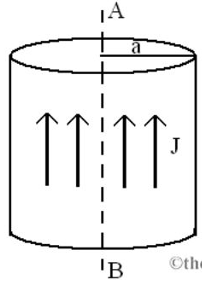# Problem: An electric current is flowing through a long cylindrical conductor with radius a = 0.15 m. The current density J= 5.5 A/m2 uniform in the cylinder. In this problem, we consider an imaginary cylinder with radius r around the axis AB (a) Express B in terms of J and r.(b) For r = 0.5a, calculate the numerical value of B in Tesla

###### FREE Expert Solution

The magnetic field at r < a:

$\overline{){\mathbf{B}}{\mathbf{=}}\frac{{\mathbf{\mu }}_{\mathbf{0}}{\mathbf{i}}_{\mathbf{r}}}{\mathbf{2}\mathbf{\pi }\mathbf{r}}}$

(a)

B = μ0(πr2J)/(2πr) = 0rJ)/2

98% (303 ratings)###### Problem Details

An electric current is flowing through a long cylindrical conductor with radius a = 0.15 m. The current density J= 5.5 A/m2 uniform in the cylinder. In this problem, we consider an imaginary cylinder with radius r around the axis AB

(a) Express B in terms of J and r.

(b) For r = 0.5a, calculate the numerical value of B in Tesla